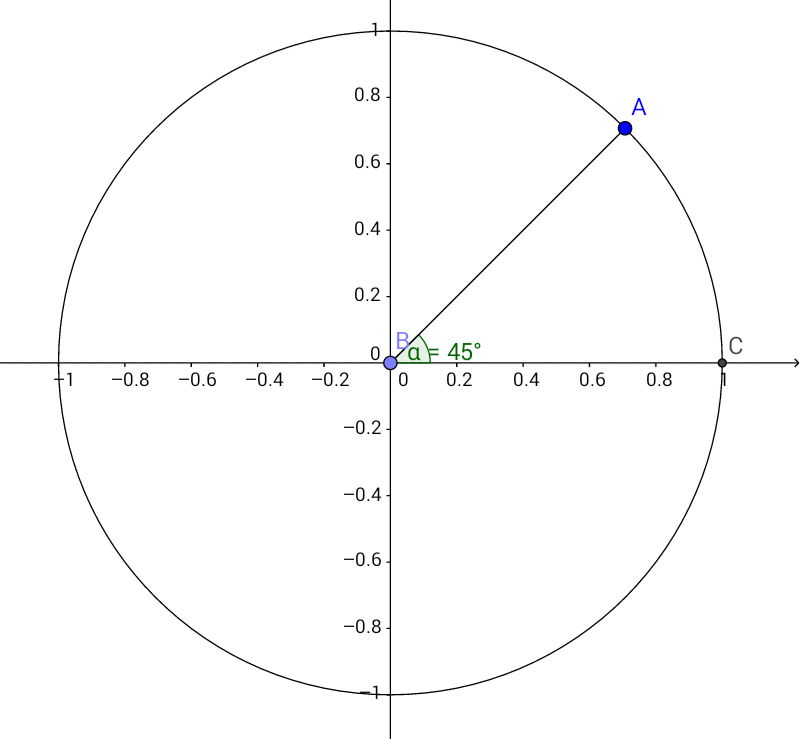So... in school we are seeing first grade equations and the polar coordinate system. We were watching how to transport one from another... and I wondered how equations looked like in the polar coordinate system. We have already seen how they look like or to interpret some of its behavior in the Cartesian Plane, but not in the polar. So my question is, how do equations look like in the polar system and how can we interpret them?

(I barely know about Calculus, which makes me feel like a moron. I am mostly self-taught but it's very hard for me so please, if you use Calculus to explain the idea be as clear as possible, I know it's a lot to ask but I have this idea and I am not really sure how to proceed)

Best regards! Have a nice day!Note by Karen Sarai Morales Montiel
3 years, 6 months ago

This discussion board is a place to discuss our Daily Challenges and the math and science related to those challenges. Explanations are more than just a solution — they should explain the steps and thinking strategies that you used to obtain the solution. Comments should further the discussion of math and science.

When posting on Brilliant:

• Use the emojis to react to an explanation, whether you're congratulating a job well done , or just really confused .
• Ask specific questions about the challenge or the steps in somebody's explanation. Well-posed questions can add a lot to the discussion, but posting "I don't understand!" doesn't help anyone.
• Try to contribute something new to the discussion, whether it is an extension, generalization or other idea related to the challenge.

MarkdownAppears as
*italics* or _italics_ italics
**bold** or __bold__ bold
- bulleted- list
• bulleted
• list
1. numbered2. list
1. numbered
2. list
Note: you must add a full line of space before and after lists for them to show up correctly
paragraph 1paragraph 2

paragraph 1

paragraph 2

[example link](https://brilliant.org)example link
> This is a quote
This is a quote
    # I indented these lines
# 4 spaces, and now they show
# up as a code block.

print "hello world"
# I indented these lines
# 4 spaces, and now they show
# up as a code block.

print "hello world"
MathAppears as
Remember to wrap math in $$ ... $$ or $ ... $ to ensure proper formatting.
2 \times 3 $2 \times 3$
2^{34} $2^{34}$
a_{i-1} $a_{i-1}$
\frac{2}{3} $\frac{2}{3}$
\sqrt{2} $\sqrt{2}$
\sum_{i=1}^3 $\sum_{i=1}^3$
\sin \theta $\sin \theta$
\boxed{123} $\boxed{123}$

Sort by:

A polar form of an equation is basically, another way to view a system of equations (a graph in the coordinate plane). Instead of viewing the graph of the equation on a sheet of paper and analysing it along the horizontal and vertical dimensions (length), we consider ourselves to be a point object located at the origin of the coordinate system and looking at the graph of the equation that will be in our plane. So, in this case, the only possible way of differentiating between two different points its the points, distance from us (origin) and the direction of that point. The direction of the point is indicated by the angle the point makes with the x-axis. So, no two distinct points can have the same set of parameters (distance and direction).

As, an example, consider the equation $x^2 + y^2 = 1$. Here is how the graph is plotted:-So, here for a particular point A, the distance parameter is the distance from the origin i.e $AB$ which happens to be $1$ here, and the direction is the angle $\angle BAC$ which here is $45°$.

- 3 years, 5 months ago

Thank you!...Although I don't see the graph...

- 3 years, 5 months ago

Refresh your page, there is one in my comment.

- 3 years, 5 months ago

Thanks!

- 3 years, 5 months ago